Linear Algebra MCQ Level - 1

# Linear Algebra MCQ Level - 1

Test Description

## 10 Questions MCQ Test Topic wise Tests for IIT JAM Physics | Linear Algebra MCQ Level - 1

Linear Algebra MCQ Level - 1 for IIT JAM 2023 is part of Topic wise Tests for IIT JAM Physics preparation. The Linear Algebra MCQ Level - 1 questions and answers have been prepared according to the IIT JAM exam syllabus.The Linear Algebra MCQ Level - 1 MCQs are made for IIT JAM 2023 Exam. Find important definitions, questions, notes, meanings, examples, exercises, MCQs and online tests for Linear Algebra MCQ Level - 1 below.
Solutions of Linear Algebra MCQ Level - 1 questions in English are available as part of our Topic wise Tests for IIT JAM Physics for IIT JAM & Linear Algebra MCQ Level - 1 solutions in Hindi for Topic wise Tests for IIT JAM Physics course. Download more important topics, notes, lectures and mock test series for IIT JAM Exam by signing up for free. Attempt Linear Algebra MCQ Level - 1 | 10 questions in 30 minutes | Mock test for IIT JAM preparation | Free important questions MCQ to study Topic wise Tests for IIT JAM Physics for IIT JAM Exam | Download free PDF with solutions
 1 Crore+ students have signed up on EduRev. Have you?
Linear Algebra MCQ Level - 1 - Question 1

### Let  A be a m × n matrix with row rank = r = column rank. The dimension of the space of solution of the system of linear equations AX = 0 is :

Detailed Solution for Linear Algebra MCQ Level - 1 - Question 1

Given that rank  A = r
⇒ There would be r  linearly independent solutions
Dim (A) = dim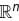– rank = n – r

The correct answer is: n – r

Linear Algebra MCQ Level - 1 - Question 2

### What would be the dimension for the general solution of the homogeneous system. x1 + 2x2 – 3x3 + 2x4 – 4x5 = 0 2x1 + 4x2 – 5x3 + x4 – 6x5 = 0 5x1 + 10x2 – 13x3 + 4x4 – 16x5 = 0

Detailed Solution for Linear Algebra MCQ Level - 1 - Question 2

Consider the coefficient matrix,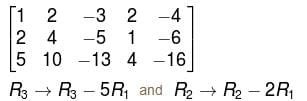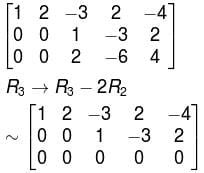The system in echelon form has three free variables,  x3x4x5
hence dim = 3

Linear Algebra MCQ Level - 1 - Question 3

### If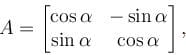then A-1 is equal to :

Detailed Solution for Linear Algebra MCQ Level - 1 - Question 3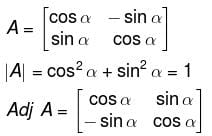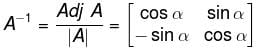The correct answer is: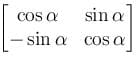Linear Algebra MCQ Level - 1 - Question 4

A matrix M has eigen values 1 and 4 with corresponding eigen vectors (1, –1)T  and  (2, 1)T, respectively. Then M  is :

Detailed Solution for Linear Algebra MCQ Level - 1 - Question 4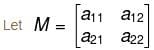We know that if λ is an eigenvalue of M, then X is the corresponding eigen vector then,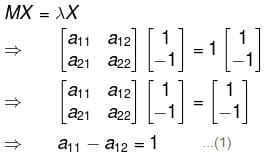a12 – a22 = –1       ...(2)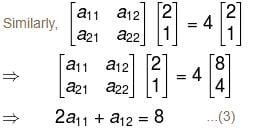2a21 + a22 = 4         ...(4)
Solving (1), (2), (3), (4), we get
a11 = 3, a12 = 2, a21 = 1, a22 = 2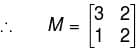The correct answer is: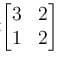Linear Algebra MCQ Level - 1 - Question 5

If rank of matrix A is 5 and nullity of A is 3, then A is of order :

Detailed Solution for Linear Algebra MCQ Level - 1 - Question 5

Rank is given by the number of non-zero rows the echelon from of the matrix and nullity is given by the Number of zero rows.

⇒   By sylvester's law, order of the matrix will be = rank + nullity
= 5 + 3
= 8

Linear Algebra MCQ Level - 1 - Question 6

The three equations,
–2x + y + z = a
x – 2y + z = b
x + y – 2z = c

will have no solution, unless :

Detailed Solution for Linear Algebra MCQ Level - 1 - Question 6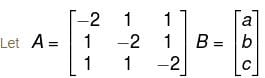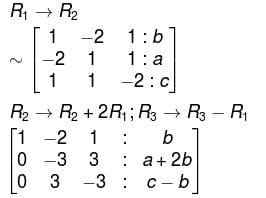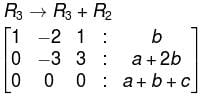Hence, the system won't contain any solution unless a + b + c becomes 0.

The correct answer is: a + b + c = 0

Linear Algebra MCQ Level - 1 - Question 7

Solving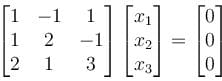will give,

Detailed Solution for Linear Algebra MCQ Level - 1 - Question 7

Consider the coefficient matrix, say  A, i.e.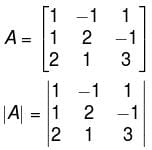= 1(6 + 1) + 1(3+2) + 1(1 – 4)

= 9 ≠ 0
Hence, rank  A = 3 = Number of unknowns.
∴ There will be only one solution of the given matrix equation and that is

x = y = z = 0.
The correct answer is: (0 0 0)T

Linear Algebra MCQ Level - 1 - Question 8

The matrix A is represented as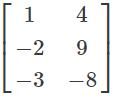. The transpose of the matrix of this matrix is represented as?

Detailed Solution for Linear Algebra MCQ Level - 1 - Question 8

Given matrix is a 3×2 matrix and the transpose of the matrix is 3 × 2 matrix. The values of matrix are not changed but the elements are interchanged, as row elements of a given matrix to the column elements of the transpose matrix and vice versa but the polarities of the elements remains same.

Linear Algebra MCQ Level - 1 - Question 9

Find the values of x, y, z and w from the below condition.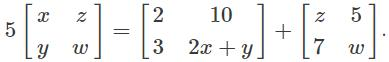Detailed Solution for Linear Algebra MCQ Level - 1 - Question 9

5z = 10 + 5 => 5z = 15 => z = 3
5x = 2 + z => 5x = 5 => x = 1
5y = 3 + 7 => 5y = 10 => y = 2
5w = 2 + 2 + w => 4w = 4 => w = 1.

Linear Algebra MCQ Level - 1 - Question 10

Let P be a matrix of order m × n and Q be a matrix of order n × p, n ≠ p. If rank (P) = n and rank of (Q) = p, then rank (PQ)  is :

Detailed Solution for Linear Algebra MCQ Level - 1 - Question 10

For the matrix P (m * n), rank<= min{ m,n}

Similarly for matrix Q (n * p), rank <= min{n , p}

Now, the rank of PQ <= min {rank of P ,rank of Q}

=> rank (PQ) <= min{ min {m,n} ,min{n,p}}

=> rank (PQ) <= min {m, n, p}

## Topic wise Tests for IIT JAM Physics

217 tests
 Use Code STAYHOME200 and get INR 200 additional OFF Use Coupon Code
Information about Linear Algebra MCQ Level - 1 Page
In this test you can find the Exam questions for Linear Algebra MCQ Level - 1 solved & explained in the simplest way possible. Besides giving Questions and answers for Linear Algebra MCQ Level - 1, EduRev gives you an ample number of Online tests for practice

217 tests